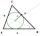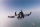Expression of a variable from formula - math word problems - page 17

1. CoefficientDetermine the coefficient of this sequence: 7.2; 2.4; 0.8
2. Inscribed circleThe circle inscribed in a triangle has a radius 3 cm. Express the area of the triangle using a, b, c.
3. Difference AP 4Calculate the difference of the AP if a1 = 0.5, a2 + a3 = -1.1
4. Volume and areaWhat is the volume of a cube which has area of 361 cm2?
5. CombinationsIf the number of elements increase by 3, it increases the number of combinations of the second class of these elements 5 times. How many are the elements?
6. Boys and girlsThere are 28 girls in the hall. 5/7 of all children are boys. How many children and how many boys are there?
7. TrapeziumThe area of trapezium is 35 cm2. Find its altitude if the bases are 6cm and 8 cm.
8. Circle chordCalculate the length of the chord of the circle with radius r = 10 cm, length of which is equal to the distance from the center of the circle.
9. The ropeA 68 centimetre long rope is used to make a rhombus on the ground. The distance between a pair of opposite side corners is 16 centimetres what is the distance between the other two corners?
10. Third memberDetermine the third member of the AP if a4=93, d=7.5.
11. AlleyAlley measured a meters. At the beginning and end are planted poplar. How many we must plant poplars to get the distance between the poplars 15 meters?
12. Three paintersThe three painters have painted bridge. The first would work done in 5 days, the second in 6 days, and the third in 7.5 days. How long will the bridge work if they work together?
13. Right triangle from axesA line segment has its ends on the coordinate axes and forms with them a triangle of area equal to 36 sq. Units . The segment passes through the point ( 5,2). What is the slope of the line segment. ?
14. Hexagon cut pyramidCalculate the volume of a regular 6-sided cut pyramid if the bottom edge is 30 cm, the top edge us 12 cm, and the side edge length is 41 cm.
15. Free fallHow long does the stone fall freely into a depth of 80m? What speed will it hit the bottom of the abyss?
16. Solve equationsolve equation: ?
17. Surface of cubesPeter molded a cuboid 2 cm, 4cm, 9cm of plasticine. Then the plasticine split into two parts in a ratio 1:8. From each part made a cube. In what ratio are the surfaces of these cubes?
18. Area of iso-trapFind the area of an isosceles trapezoid, if the lengths of its bases are 16 cm, and 30 cm, and the diagonals are perpendicular to each other.
19. Diagonalhe rectangular ABCD trapeze, whose AD arm is perpendicular to the AB and CD bases, has area 15cm square. Bases have lengths AB = 6cm, CD = 4cm. Calculate the length of the AC diagonal.
20. Trapezoid - intersection of diagonalsIn the ABCD trapezoid is AB = 8 cm long, trapezium height 6 cm, and distance of diagonals intersection from AB is 4 cm. Calculate trapezoid area.

Do you have an interesting mathematical word problem that you can't solve it? Enter it, and we can try to solve it.

To this e-mail address, we will reply solution; solved examples are also published here. Please enter e-mail correctly and check whether you don't have a full mailbox.